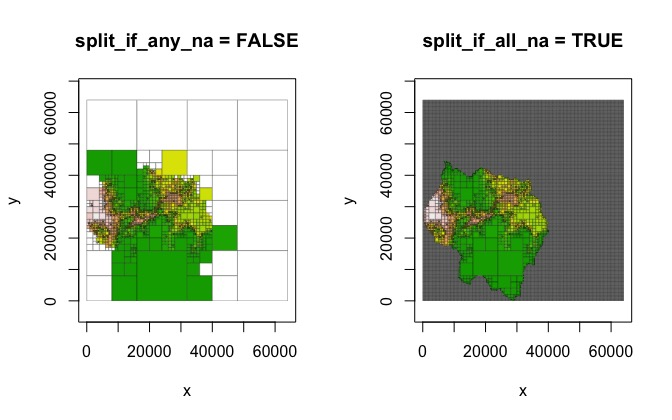Vignette content

This vignette goes over the process of creating a quadtree and covers all of the various parameter settings that can be used to modify the way a quadtree is created. Note that all the examples use the following example raster:

library(raster)

data(habitat)
rast <- habitat
plot(rast, main = "sample raster")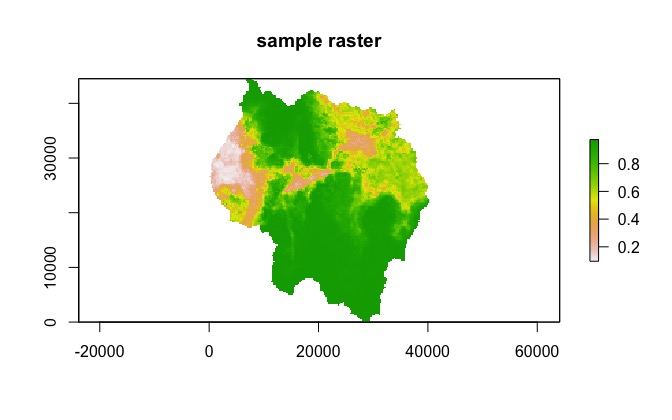Overview of the quadtree data structure

Quadtrees are tree data structures where each node is allowed to have either zero or four children. This data structure can be used to represent two-dimensional space by interpreting each node as a rectangular cell. The root of the tree represents a single cell that encompasses the entire area. If a node has children, they represent the four cells created when the node is divided into quadrants. This creates a hierarchical data structure where any given point in space is contained within many different cells at different levels. In order to represent the value of some variable across space, each node has a value that represents the value of that variable in the region covered by the node. This means that any point may be associated with multiple values - this can be useful in some situations. However, it may also be desirable for each point to be associated with only a single value. The terminal nodes can be treated as the only value for any given point, and the values at the higher levels can essentially be ignored. This creates a space-exhausting surface like a raster where every point in space has a single value. Unlike a raster, however, the hierarchical nature of a quadtree easily facilitates variable-sized cells. When a quadtree is used in this fashion it is referred to as a region quadtree.

This feature of quadtrees make them advantageous in certain situations. In some applications it may be critical for the data structure to have a fine resolution in certain areas, while other areas may be suitably represented by large cells. For example, quadtrees have been used for image compression - by allowing largely homogeneous areas of an image to be represented by large cells, the amount of data needed to represent the image can be greatly reduced while still preserving fine resolution in heterogeneous areas.

The following figure shows a quadtree data structure (left), it’s spatial representation (middle), and a 3D plot showing how the data structure and the spatial representation correspond (right).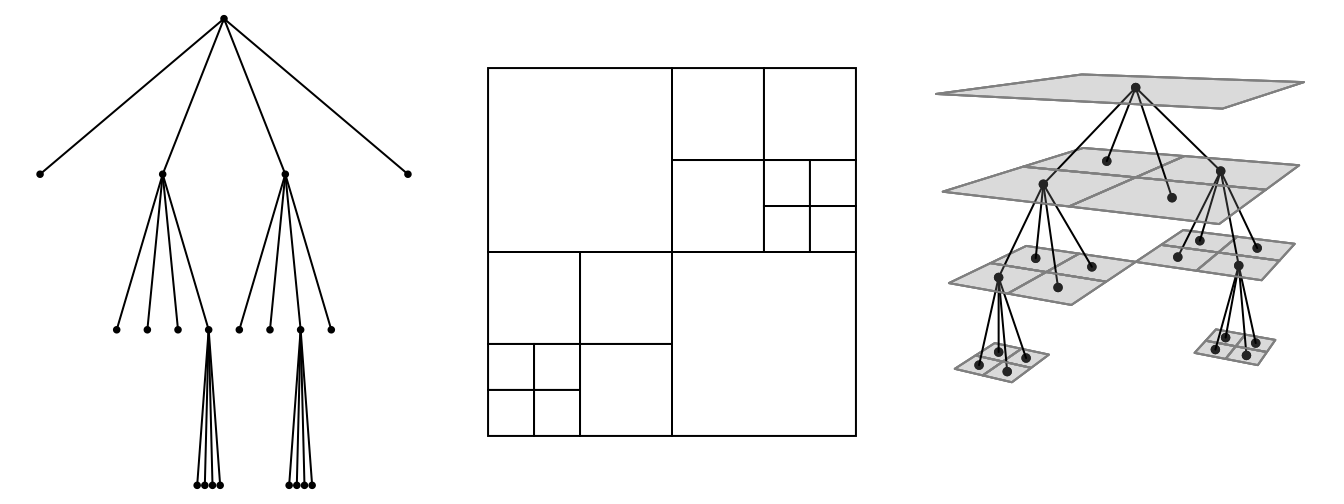In the quadtree package, a quadtree is created from a raster or a matrix by successively dividing the raster/matrix into smaller and smaller cells, with the decision on whether to divide a quadrant determined by a function that checks the cell values within each quadrant and returns TRUE if it should be split, and FALSE otherwise. Initially, all of the cells in the raster are considered. If the cell values meet the condition determined by the splitting function, the raster is divided into four quadrants - otherwise, the raster is not divided further and the value of this larger cell is calculated by applying a ‘combine function’ that aggregates the cell values into a single value (for example, mean or median). If a quadrant is split, the process is repeated for each of those ‘child’ quadrants, and then for their children, and so on and so forth, until either the split function returns FALSE or the smallest possible cell size (as determined by the input raster) has been reached.

If a given quadrant has dimensions that are not divisible by two (for example, 5 x 5), then the process stops. Because of this, only rasters that have dimensions that are a power of two can be divided down to their smallest cell size. In addition, only square rasters can be divided down to their smallest cell size. To create quadtrees from rasters that have dimensions that are not a power of two and are not square, two options are provided. The choice of method is determined by the adj_type parameter.

The "expand" method

In the "expand" method, NA cells are added to the raster in order to create an expanded raster whose dimensions are a power of two. The smallest number that is a power of two but greater than the larger dimension is used as the dimensions of the expanded raster. In the following example, the raster has dimensions of 178 x 161. To make it suitable for quadtree creation, NA rows and columns are added in order to create a raster with dimensions 256 x 256 (as 256 is the smallest power of two that is also greater than 178), and then the quadtree is created from that raster.

dim(rast) # not a power of 2
#>  178 161   1
plot(qt, border_lwd = .3, main = "expand raster dimensions")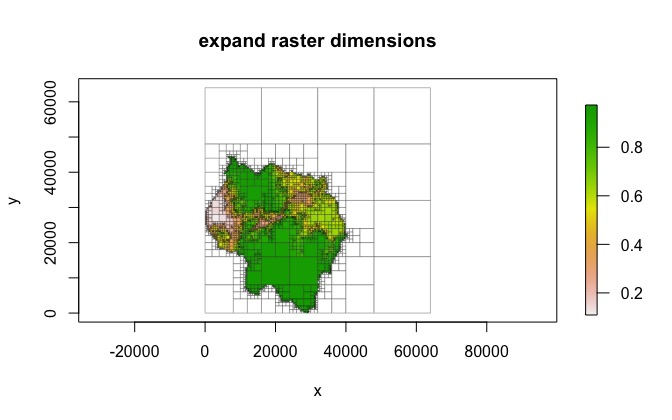The "resample" method

In the "resample" method, the raster is resampled in order to create a square raster with dimensions that are a power of two. If the data does not have the same number of rows and columns, resampling the raster to have an equal number of rows and column will result in rectangular but non-square cells. This resampled raster is then used to create the quadtree.

plot(qt, border_lwd = .3, main = "resample (without NA padding)")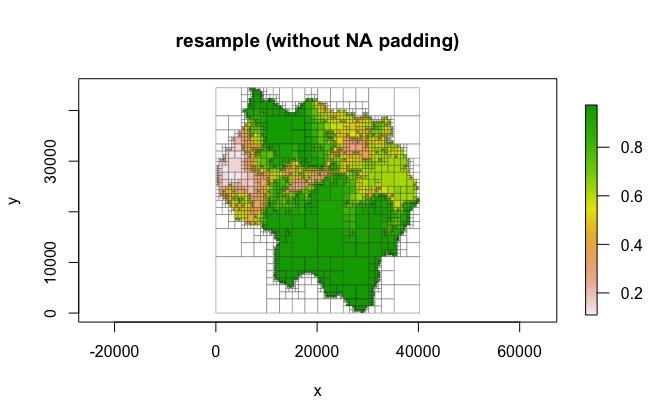If square cells are desired, an additional step is added to make the raster square by setting resample_pad_nas to be TRUE (the default). This is done in a way similar to the method described above. The smaller dimension is padded with NA cells in order to equal the larger dimension. As stated previously, our sample raster has dimensions 178 x 161, so NA columns are added in order to create a raster with dimensions 178 x 178. Then, the raster is resampled to a user-specified dimension (determined by the resample_n_side parameter). For example, the user could set resample_n_side to be 128, which will resample the 178 x 178 raster to 128 x 128. This raster can then be used to create a quadtree.

plot(qt, border_lwd = .3, main = "resample (with NA padding)")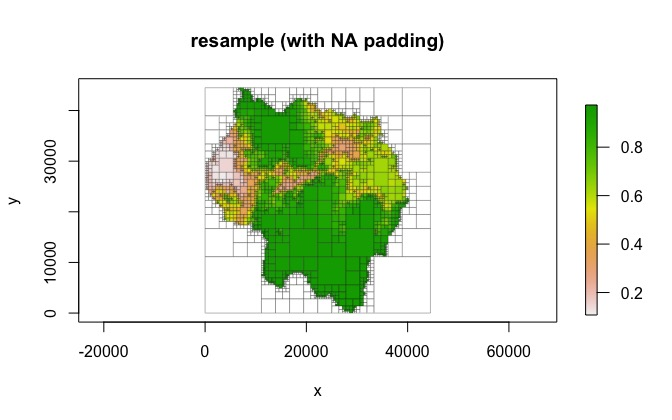If adj_type is "none", the provided matrix/raster is used ‘as is’, with no dimension adjustment. In this case, doing so results in a single cell quadtree, since 161 (the number of columns) is not divisible by two.

plot(qt, main = "adj_type = 'none'")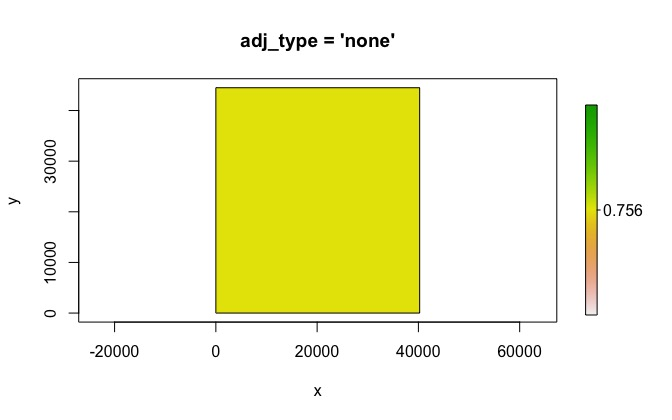Splitting and aggregating functions

The method used to determine whether or not to split a cell as well as the method used to aggregate cell values can be defined by the user. Simple methods are already provided, but custom functions can be defined.

Default methods

Splitting functions

For splitting a quadrant, two methods are provided. "range" checks the difference between the minimum value and the maximum value within the quadrant - if this difference exceeds split_threshold, the quadrant is split. "sd" uses the standard deviation of the cell values within a quadrant - if the standard deviation exceeds split_threshold, the quadrant is split.

qt_range <- quadtree(rast, .1, split_method = "range")
qt_sd <- quadtree(rast, .1, split_method = "sd")

par(mfrow = c(1, 2))
plot(qt_range, crop = TRUE, na_col = NULL, zlim = c(0, 1), border_lwd = .3,
legend = FALSE, main = "split_method = 'range'")
plot(qt_sd, crop = TRUE, na_col = NULL, zlim = c(0,1), border_lwd = .3,
legend = FALSE, main = "split_method = 'sd'")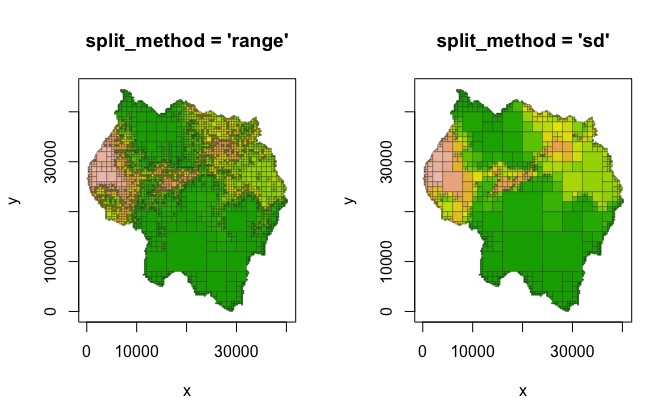Combine functions

Four methods to aggregate cell values are provided - "mean", "median", "min", and "max" - the names are self-explanatory. Note that in the following example the structures of the quadtrees are identical - the combine function has no influence on the decision to split a cell.

qt_mean <- quadtree(rast, .1, "sd", combine_method = "mean")
qt_median <- quadtree(rast, .1, "sd", combine_method = "median")
qt_min <- quadtree(rast, .1, "sd", combine_method = "min")
qt_max <- quadtree(rast, .1, "sd", combine_method = "max")

par(mfrow = c(2, 2), mar = c(.5, .5, .5, .5))
plot(qt_mean, crop = TRUE, na_col = NULL, axes = FALSE, legend = TRUE,
border_lwd = .3, zlim = c(0,1), main = "mean")
plot(qt_median, crop = TRUE, na_col = NULL, axes = FALSE, legend = TRUE,
border_lwd = .3, zlim = c(0,1), main = "median")
plot(qt_min, crop = TRUE, na_col = NULL, axes = FALSE, legend = TRUE,
border_lwd = .3, zlim = c(0,1), main = "min")
plot(qt_max, crop = TRUE, na_col = NULL, axes = FALSE, legend = TRUE,
border_lwd = .3, zlim = c(0,1), main = "max")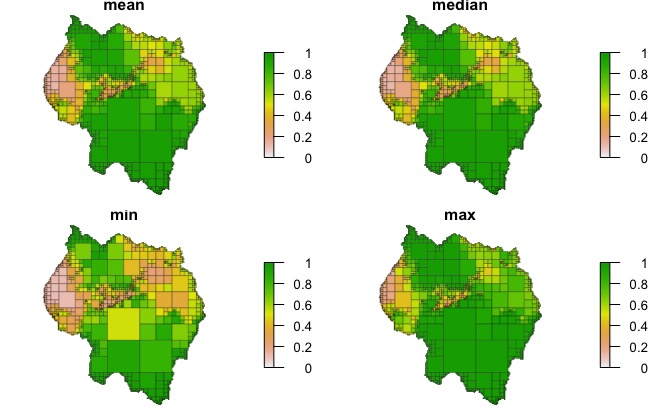Custom methods

Splitting functions

Custom functions can be written to apply more complex rules to splitting and combining. These functions must take two parameters: vals and args. vals is a numeric vector of the values of the cells within the current quadrant. args is a named list that contains the arguments needed by the custom function. Any parameters needed for the function should be accessed through args. Note that even if no extra parameters are needed, the custom function still needs to take an args parameter - in that case it just won’t be used by the function.

split_fun must return a boolean, where TRUE indicates that the quadrant should be split. An important note to make is that any custom function must be able to handle NA values. The function must always return either TRUE or FALSE - if NA is ever returned an error will occur.

For example, a simple splitting function that splits a quadrant when any of the values are below a given limit could be defined as follows:

split_fun <- function(vals, args) {
return(any(vals < args$threshold)) } We can then use this with the quadtree() function to apply the splitting method. Note that because the function makes use of an element of args named threshold, the split_args parameter needs to contain an element called threshold: qt <- quadtree(rast, split_method = "custom", split_fun = split_fun, split_args = list(threshold = .8)) plot(qt, crop = TRUE, na_col = NULL, border_lwd = .3, main = "custom splitting function")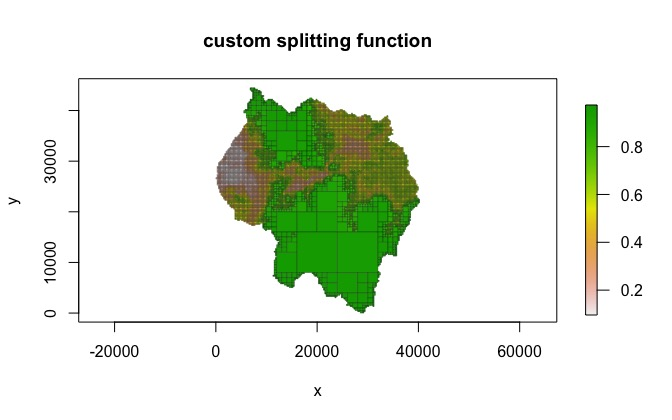Combine functions As with split_fun, combine_fun must take two arguments named vals and args. Custom combine functions must return a single numeric value, and unlike split_fun, combine_fun is allowed to return NA values. For example, the following function can be used to create a binary quadtree which only takes on the values 0 and 1, based on some cutoff threshold. cmb_fun <- function(vals, args) { if (any(is.na(vals))) { return(NA) } if (mean(vals) < args$threshold) {
return(0)
} else {
return(1)
}
}

qt <- quadtree(rast, .1, combine_method = "custom", combine_fun = cmb_fun,
combine_args = list(threshold = .5))
plot(qt, crop = TRUE, na_col = NULL, border_lwd = .3,
main = "custom combine function")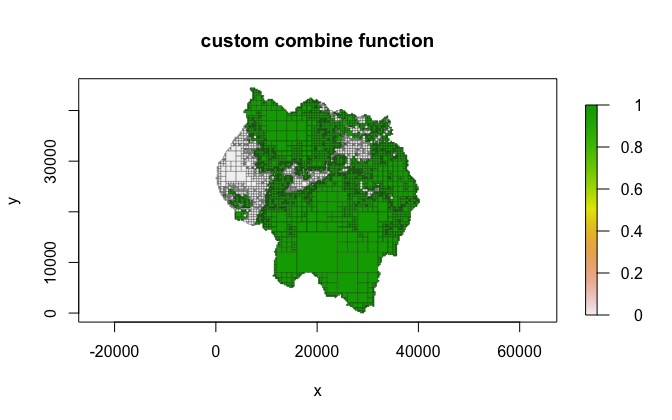Creating a quadtree using a template

In the following example, a raster representing the presence/absence of roads is used to create a quadtree, and then that quadtree is used as a template to create a quadtree from a different raster.

# use a custom function so that a quadrant is split if it contains any 1's
split_if_one <- function(vals, args) {
if(any(vals == 1, na.rm = TRUE)) return(TRUE)
return(FALSE)
}
qt_template <- quadtree(template, split_method = "custom",
split_fun = split_if_one)

# now use the template to create a quadtree from 'rast'

par(mfrow = c(1, 3), mar = c(0,0,3,0))
plot(template, axes = FALSE, box = FALSE, legend = FALSE,
main = "template raster")
plot(qt_template, crop = TRUE, na_col = NULL, border_lwd = .3 ,axes = FALSE,
legend = FALSE, main = "template quadtree")
plot(qt, crop = TRUE, na_col = NULL, border_lwd = .3, axes = FALSE,
legend = FALSE, main = "quadtree created using template")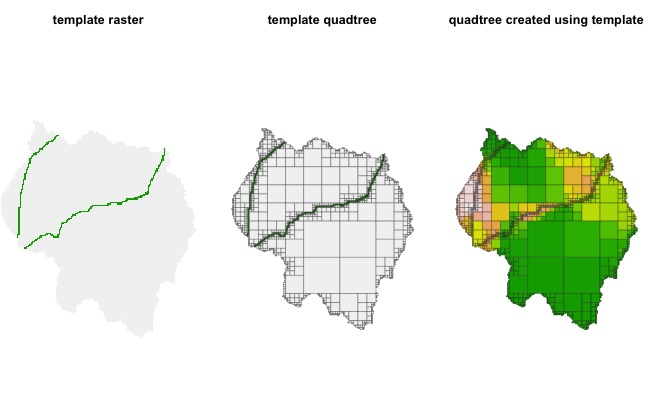Other parameters

max_cell_length and min_cell_length

The max_cell_length and min_cell_length parameters let the user specify the range of allowable cell sizes. If max_cell_length is not NULL, then the maximum cell size in the resulting quadtree will be max_cell_length. This essentially forces any quadrants larger than max_cell_length to split. The one exception is that a quadrant that contains entirely NA values will not be split. Similarly, the min_cell_length parameter can be used to define a minimum side length for all cells, such that a quadrant cannot be split if its children would be smaller than min_cell_length.

qt_max_cell <- quadtree(rast, .15, max_cell_length = 1000)
qt_min_cell <- quadtree(rast, .15, min_cell_length = 1000)

par(mfrow = c(1, 2))
plot(qt_max_cell, crop = TRUE, na_col = NULL, border_lwd = .3, legend = FALSE,
main = "max_cell_length = 1000")
plot(qt_min_cell, crop = TRUE, na_col = NULL, border_lwd = .3, legend = FALSE,
main = "min_cell_length = 1000")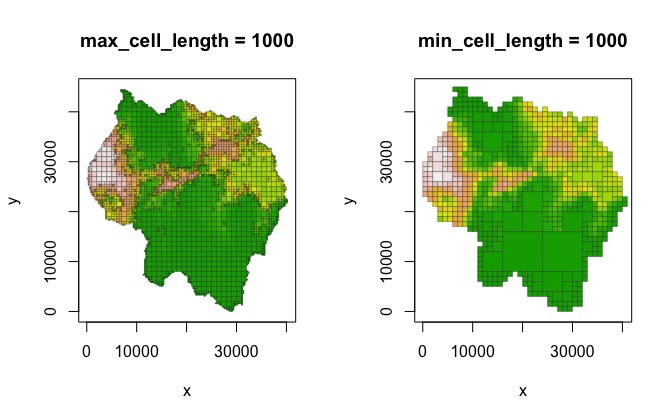split_if_any_na and split_if_all_na

The split_if_any_na and split_if_all_na parameters control how NA values are handled. If split_if_any_na is TRUE (the default), a quadrant will be split if any of the values are NA. This ensures that rasters with irregular shapes maintain their shape in the resulting quadtree representation. If FALSE, quadrants with NAs are not automatically split - note that this can produce unexpected results if the raster is irregularly shaped (see the example below). split_if_all_na controls what happens when a quadrant consists entirely of NA values. If FALSE (the default), these quadrants are not split. If TRUE, these quadrants are automatically split, which results in quadrants with all NA values being split to the smallest possible cell size.

qt_any <- quadtree(rast, .15, split_if_any_na = FALSE)
qt_all <- quadtree(rast, .15, split_if_all_na = TRUE)

par(mfrow = c(1, 2))
plot(qt_any, border_lwd = .3, legend = FALSE, main = "split_if_any_na = FALSE")
plot(qt_all, border_lwd = .3, legend = FALSE, main = "split_if_all_na = TRUE")